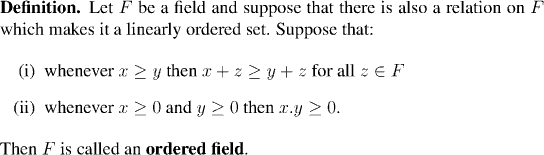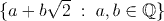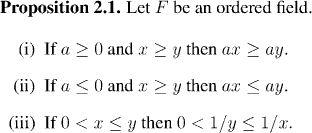Analysis WebNotes
arrow_back arrow_forward

# Class Contents

## Ordered Fields

Recall: Our intuition is that the real numbers are a field and a linearly ordered set. Just as the distributive law links together the two operations of multiplication and addition, we need rules which tell us how multiplication and addition affect the ordering of elements of our field. As long as the axioms of the linear order and the arithmetic operations are independent, we can't expect to be able to deduce any connection. But if we take two simple rules as additional axioms, then all the other rules we need for manipulating inequalities will follow from them.Example:Example:

The set of real numbers:is a field. (see note)

Example:

{0,1} is not an ordered field with the order 0< 1.

We state here for the record:

First Axiom of the Real Numbers: The real numbers are an ordered field.

As we remarked above, all the algebraic properties of inequalities that we need can be deduced from the axioms for an ordered field (usually from a combination of the rules for field, for an ordered set, and for the two additional axioms for ordered fields).Factoid:

1. If F is an ordered field then the square of any element is non-negative. (ie greater than or equal to zero). In particular, 1> 0.
2. If x> 0 then -x< 0, in any ordered field.

Factoid:

Every ordered field is an infinite set. In fact, every ordered field contains an isomorphic copy of the rational numbers.

The remaining axiom of the real numbers (there is only one more) is entirely to do with the way the real numbers are ordered. So we now forget about the arithmetic properties of the reals for a while, and concentrate on linearly ordered sets in the next section.